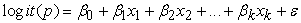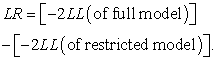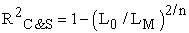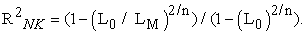﻿ Statistical Modelling of Categorical Outcome with More than Two Nominal CategoriesPublications are Open
Access in this journal
Article Versions
Export Article
• Normal Style
• MLA Style
• APA Style
• Chicago Style
Research Article
Open Access Peer-reviewed

### Statistical Modelling of Categorical Outcome with More than Two Nominal Categories

Fatma D.M. Abdallah
American Journal of Applied Mathematics and Statistics. 2018, 6(6), 262-265. DOI: 10.12691/ajams-6-6-7
Received August 13, 2018; Revised October 04, 2018; Accepted December 04, 2018

### Abstract

This paper aims to explain and apply an important statistical method used for modelling categorical outcome variable with at least two unordered categories. Logistic regression model especially multinomial logistic type (MNL) model is the best choice to model unordered qualitative data. A simulation study was done to examine the efficiency of the model in representing categorical response variable. Three explanatory variables (age, species, and sex) are used for discrimination. While the outcome variable was Rose Bengal Plate Test (RBPT) results which has four outcome categories (negative, positive, false positive, and false negative). Therefore, logit model will be utilized to model this data. MNL models were fitted using SPSS packages and parameters estimated depending on maximum likelihood (MLE) by the Newton-Raphson algorithm. This model depends mainly on two estimates to interpret the results, they are the regression coefficient and the exponentiated coefficients which known as the odds ratio. This model was a good fitted for description the data of 500 values of Rose Bengal Plate Test results of Brucella in sheep and goat species. The results showed fitting of the model to the data with highly significant likelihood ratio statistic for the overall model (P value = 0.000**). Wald test was significant for all variables in positive category and this indicated that age, species and sex are good predictors for test results. The odds ratio in case of positive category for age, species and sex was 1.589, 0.214 and 0.133 respectively.

### 1. Introduction

Different statistical techniques for modelling of categorical data have increased in last years. Many traditional methods were used for this purpose such as Chi square and log-linear models. It is believed that the logistic models are convenient for representing and predicting categorical data. Multinomial regression logistic models are types of logistic regression models and considered an extension of binary logistic regression methods that can be used in this area of statistics.

In the health sciences, the model is known as polychotomous logistic regression and in econometrics as the discrete choice model 1.

It is a non-linear S-shaped distribution function, and can be utilized in many implementations 2. So the estimated probabilities of the logit distribution are between 0.0 and 1.0. The variation between the logistic function and the linear regression function is that, the values of the first one falls between zero and one, whereas the second one values are absolute.

Multinomial regression models are used to analyze data where the outcome variable is categorical with at least two unordered categories while the predictor variables could be continuous, categorical variables, or both 1.

These models do not require the known assumptions of any model such as normality, linearity and the variances homogeneity for continuous data with exception of multicollinearity 3, so these models can be used in many fields with major advantage.

Fitting the model to the data depending on two types of measures: predictive power measures such as pseudo R2 and goodness of fit tests such as deviance and Pearson chi-square. It’s common for models with high R2 to give acceptable goodness of fit tests. But, models with very small R2, may fit the observed data very well.

In order to measure the action of any explanatory variable on the outcome one, the test statistic is the likelihood ratio (LR). If this probability ratio is significant for the overall model, this means that the explanatory variables have participated in the forecasting of the resultant one. If the outcome variable has K groups, then the coefficients (βn) associated with each explanatory variable Xn are K − 1 4.

The beta coefficient statistic and the odd ratios (the exponentiated coefficients) can explain the results of the model under study. Positive beta coefficients, increases in predictor values leads to an increase of probability in the higher-numbered response categories 5, 6, 7.

### 2. Materials and Methods

A simulation study was done to explore the model efficiency in predicting categorical nominal response variables.

The dependent or the outcome variable (RBPT results) contain four groups (negative, positive, false positive and false negative).It is unordered categories therefore the model which will be applied is the multinomial logistic regression (MNL). It is important to select what is called reference category (negative category is the reference one). Then the model compares all the groups of the outcome variable with this reference one. This category considered as the comparison point for all analyses 5.

RBPT is a serological test where the samples of serum and RBPT antigen were thoroughly mixed and reading was done within 4 minutes to detect the results.

The independent or explanatory variables are: age which is considered quantitative and species (sheep and goat), and sex. Species and sex are considered categorical.

2.1. The Model Assumptions

• The independent variable may either be quantitative or qualitative.

• The outcome variable is qualitative which divided into different unordered categories.

• The data do not require to have a normal distribution, no linear relationship and no equality of variances.

2.2. The Mathematical Model

The logistic function according to 2 is(1)

where,

p: the chance of choosing a category of RBPT results,

X1,…, Xk: the predictor variables k,

β0: the intercept,

β1, …, βk: the parameter estimates on the explanatory variables Xk.

ε: error terms.

The model parameters produced depending on estimation of maximum likelihood (MLE) of the model. To get the maximum likelihood estimate ofa Newton-Raphson iterative estimation method is used. SPSS packages version 20 8 is used to execute the analysis process.

2.3. -2 log likelihood

The -2 log likelihood has a chi-square distribution, which applied to show whether the test result is significant.(2)

The LR statistic of the full model includes all of the explanatory factors and producing LRF. The LR statistic of the reduced model excluded the explanatory factors xn and producing LRR.

2.4. R square Measures or Pseudo R2

In the ordinary linear regression there is no pseudo R2. The goodness of fit measure (R2) is as follows:(3)

Where b is the regression coefficient, x is the independent variable and y is the dependent one.

In this model, several pseudo R2 have been developed due to the lack of statistic equivalent to R2 .They are called “pseudo” R-squares as their values, lying between 0 to 1, so they are similar to R2 9.

There are some of the common pseudo R2:

1. McFadden’s R2, which is shown as in the following equation 10.(4)

where L0 means the likelihood function in a model without predictor variables, and LM means the likelihood for the estimated model. Ln(L0) is similar to the residual sum of squares in linear regression model.

2. The Cox and Snell R2 which is as in this equation 11.(5)

where n is the number of observations. This measure shows the efficiency of the full model over the intercept model.

3. The Nagelkerke R2 is defined as 12.(6)

These tests are a part of goodness of fit statistics. Goodness of fit its meaning is that the model convenient for the data. Any model fits the data poorly if its residual variation is large 13.

2.5. Wald Test

It is a statistical measure to test if each variable in the model is significant or not.

2.6. Odds Ratio

The odds ratio is a method of comparison between two categories to show whether the probability of a certain event is similar between them 2 and 5. It is used as a statistic in the logistic regression instead of regression coefficients of the linear one to explain the prediction results.

It is the exponentiation of the multinomial logit coefficients (e(β)). The number of multinomial logistic regression models are (M − 1), where M is the number of groups of the outcome variable, and with consideration of the referent group.

β1 is the probability of transformation in the reference category versus the transformation in the comparison category as the explanatory variable changes.

### 3. Results and Discussion

The result of -2 log likelihood chi-square of overall model was 325.318 showed P value = 0.000 meaning that the difference was highly significant, so the covariates have a significant effect on the RBPT results as shown in Table 1.

The final row showed that the added variables develop the model in comparison with to the intercept only (with no added variables). The significance column (p = 0.000) denotes that the full model more efficient in predicting the outcome variable than the intercept-only model alone.

Thus the null hypothesis which stated that the difference is absent between the model without explanatory variables and the model with explanatory variables was not accepted and this indicated that the relationship between the explanatory variables and the outcome variable is present, hence accepting the alternative hypothesis.

There are some pseudo R2 measures in MNL model, as presented in Table 2.

Cox & Snell R2, the Nagelkerke R2 and McFadden R2 values indicate the amount of variation in the outcome variable and described as pseudo R2. Their values are 0.113, 0.170 and 0.109 respectively, suggesting that 11.3%, 17 % and 10.9% of the variability is explained by this set of variables included in the model.

The relationship between predictor and outcome variables depends mainly on two types of tests. The first is likelihood ratio test which determines the overall relationship between an explanatory variable and outcomes. The second is the Wald test which determines whether or not the explanatory variable is statistically significant in differentiating between two categories in each of binary logistic comparisons. If an explanatory variable has an overall relationship to the outcome variable, it does not necessarily suggest statistical significance. In fact, it may or may not be significant in differentiating between pairs of categories defined by the outcome variable.

Table 3 shows which of the predictors are statistically significant. Age, species and sex were significant because their p values are (0.033, 0.000 and 0.000) respectively.

Table 4 shows the parameter estimates (the model coefficients). There is a regression coefficient for each explanatory variable for each RBPT outcome variable category. There were four categories of the outcome, so there are three sets of logistic regression model coefficients. The first group of coefficients are belonging to the "positive" cell (which showing the comparison of the positive result group to the reference group, (negative)). The second group of coefficients are belonging to the "false positive" row (which showing the comparison of the false positive result group to the reference (negative) group. The third group of coefficients are belonging to the "false negative" row (which showing the comparison of the false negative result group to the reference (negative) group).

It is noticed that age was statistically significant for positive category as its p value was 0.022 and it was non-significant in case of false positive and false negative categories as the p value was 0.815 and 0.153 respectively.

Species was significant for positive and false positive categories as the p value was 0.008 and 0.009 respectively. It was not significant in case of false negative category as p value was 0.338.

Sex was significant for positive and false negative categories as the p value was 0.000 and 0.001 respectively. It was not significant in case of false positive category as p value was 0.454.

As mentioned before that the coefficients are exponentiated "Exp (β)" to interpret of the results.

Higher odd ratios than 1.0, mean that the predictor variables are positively related with the resultant one and lower odd ratios than 1.0, then the predictor variables are negatively related with the resultant one.

In case of age, the odd ratio is larger than 1.0 (1.589), indicating the positive contributing of this predictor to the RBPT results at positive category and its effect could increase by a value of 1.589 while the other factors are constant.

For species, the odd ratio is less than 1.0 (0.214), which indicates the negative contributing of this predictor to the RBPT results at positive level, and its effect could be predicted to decline by a value of 0.214 with fixation of the rest factors.

For sex, the odd ratio is lower than 1.0 (0.133), which indicates the negative contributing of this predictor to the

RBPT results at this level (positive), and its effect could be predicted to decrease by a value of 0.133 while the rest variables in the model are fixed as shown in Table 4.

In case of age the odd ratio is less than 1.0 (0.964), which indicates the negative contributing of this predictor to the RBPT result at false positive level. Its effect could be predicted to decline by a value of 0.964 while the other factors are held constant.

When checking species variable in the RBPT results of the level of false positive category, the odd ratio is higher than 1.0 (3.882), which indicates the positive contributing of this predictor to the RBPT results at this level, and its effect could be predicted to increase by a value of 3.882 with fixation the rest variables in the model.

For sex, the odd ratio is lower than 1.0 (0.733), which indicates the negative contributing of this predictor to the RBPT results at this level (false positive), and its effect would be predicted to decline by a factor of 0.733 with fixation the other factors of the model.

In case of age the odd ratio is less than 1.0 (0.777), which indicates the negative contributing of this predictor to the RBPT result at false negative level. Its effect could be expected to decline by a factor of 0.777 and the other factors are held constant.

When checking species predictor in the RBPT results of the level of false negative result, the odd ratio is less than 1.0 (0.628), which indicates the negative contributing of this predictor to the RBPT results at this level, its effect would be predicted to decrease by a factor of 0.628.

For sex predictor in the RBPT results of the level of false negative result, the odd ratio is smaller than 1.0 (0.152), which indicates the negative contributing of this predictor to the RBPT results at this level (false negative) as in Table 4.

### 4. Conclusion

This work used multinomial logistic regression (MNL) (logit type) to detect the associations between the Rose Bengal Plate Test results which have four categories and the independent variables. The categories of the resultant variable (negative, positive, false positive and false negative) are considered nominal (cannot be ordered). This study examined using of three risk factors in RBPT results modeling. The statistic which used here was the odds ratio as a powerful measure for interpreting the prediction results instead of regression coefficients. The pseudo R2 is used as a powerful goodness of fits statistic instead of known measures of fitness such as the deviance measure.

### References

  Hosmer, D.W. and Lemeshow, S, Applied logistic regression, Wiley-Interscience, US, 2000. In article View Article  PubMed  Judge, G., Griffiths, W.E., Hill, R.C., Lutkepohl, H. and Lee, T.C, The theory and practice of econometrics, 2nd Edition, Wiley, New York, 1985. In article  Tabachnick, B.G. and Fidell, L.S. and Osterlind, S.J, Using multivariate statistics, Allyn and Bacon Boston, US, 2001. In article PubMed  Abdulhafedh A, “Incorporating the Multinomial Logistic Regression in Vehicle Crash Severity Modeling: A Detailed Overview,” Journal of Transportation Technologies, 7:279-303. 2017. In article View Article  Greene, W, Econometric analysis, 7th Edition, Prentice Hall, Upper Saddle River, 2012. In article  Baltagi, B.H, Econometrics, 5th Edition, Springer, Berlin, 2011. In article View Article  Kleinbaum, D.G. and Klein, M, Logistic Regression: A Self-Learning Text, 3rd Edition, Springer, New York, 2010. In article View Article  SPSS, “Statistical Package for Social Sciences,” Release 20.0 versions. SPSS Inc. USA, 2006. In article  Menard, S, Applied Logistic Regression Analysis, Sage Publications, Thousand Oaks, 2002. In article View Article  McFadden, D, Conditional logit analysis of qualitative choice behavior. Frontiers in econometrics, 1974. In article  Cox, D.R. and Snell, E.J, Analysis of Binary Data, Chapman & Hall, London, 1989. In article  Nagelkerke, N.J.D, “A Note on a General Definition of the Coefficient of Determination,” Biometrika, 78: 691-692. 1991. In article View Article  Hosmer, D.W., hosmer, T., le Cessie, S., and Lemeshow, S, “A comparison of goodness-of-fit tests for logistic regression model. Statistics in medicine,” 16 (9). 965-80. 1997. In article View Article

Published with license by Science and Education Publishing, Copyright © 2018 Fatma D.M. AbdallahThis work is licensed under a Creative Commons Attribution 4.0 International License. To view a copy of this license, visit http://creativecommons.org/licenses/by/4.0/